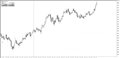Candle color inconsistency bug21

There's a bug in the indicator I made where most of the times I add the indicator to the graph, the candle colors displayed aren't correct.

The bug can be seen in the video below (the second time I add the indicator the colors are right):

https://streamable.com/9pvqf

Code:

#property indicator_buffers 7
#property indicator_plots 2

#property indicator_label1 "Candles"
#property indicator_color1 Green, Red
#property indicator_type1 DRAW_COLOR_CANDLES

#property indicator_label2 "MA"
#property indicator_color2 Green, Red, Yellow
#property indicator_type2 DRAW_COLOR_LINE
#property indicator_width2 2

double buffer_open[], buffer_high[], buffer_low[], buffer_close[];
double candle_color[];

int MA;
double buffer_ma[];
double ma_color[];

int OnInit() {
SetIndexBuffer(0, buffer_open, INDICATOR_DATA);
SetIndexBuffer(1, buffer_high, INDICATOR_DATA);
SetIndexBuffer(2, buffer_low, INDICATOR_DATA);
SetIndexBuffer(3, buffer_close, INDICATOR_DATA);
SetIndexBuffer(4, candle_color, INDICATOR_COLOR_INDEX);

SetIndexBuffer(5, buffer_ma, INDICATOR_DATA);
SetIndexBuffer(6, ma_color, INDICATOR_COLOR_INDEX);

MA = iMA(_Symbol, _Period, 20, 0, MODE_SMA, PRICE_CLOSE);

return(0);
}

int OnCalculate(const int rates_total,
const int prev_calculated,
const datetime &time[],
const double &open[],
const double &high[],
const double &low[],
const double &close[],
const long &tick_volume[],
const long &volume[],
const int &spread[]) {
int start;
if (prev_calculated == 0) {
start = 1;
} else {
start = prev_calculated - 1;
}

CopyBuffer(MA, 0, 0, rates_total, buffer_ma);

for (int i = start; i < rates_total; i++) {
if (buffer_ma[i] > buffer_ma[i - 1]) {
ma_color[i] = 0;
} else if (buffer_ma[i] < buffer_ma[i - 1]) {
ma_color[i] = 1;
} else {
ma_color[i] = 2;
}

buffer_open[i] = open[i];
buffer_high[i] = high[i];
buffer_low[i] = low[i];
buffer_close[i] = close[i];

if (close[i] > close[i - 1] && buffer_ma[i] > buffer_ma[i - 1]) {
candle_color[i] = 0;
} else if (close[i] < close[i - 1] && buffer_ma[i] < buffer_ma[i - 1]) {
candle_color[i] = 1;
}
}

return(rates_total);
}O2caJbYiGY.mp4 - Streamable
• streamable.com
Check out this video on Streamable using your phone, tablet or desktop.19815

Iervolino: the candle colors displayed aren't correct
if (close[i] > close[i - 1] && buffer_ma[i] > buffer_ma[i - 1]) {
candle_color[i] = 0;
} else if (close[i] < close[i - 1] && buffer_ma[i] < buffer_ma[i - 1]) {
candle_color[i] = 1;
}
else                            What color do you set here?

// Your code (above) code rewritten for clarity (below:)

if (buffer_ma[i] > buffer_ma[i - 1]) {
if (close[i] > close[i - 1]) candle_color[i] = 0;
else                         What color do you set here?
} else {
if (close[i] < close[i - 1]) candle_color[i] = 1;
else                         what color do you set here?
}21

whroeder1:

Thank you!

Is it correct now?

int OnCalculate(const int rates_total,
const int prev_calculated,
const datetime &time[],
const double &open[],
const double &high[],
const double &low[],
const double &close[],
const long &tick_volume[],
const long &volume[],
const int &spread[]) {
int start;
if (prev_calculated == 0) {
start = 1;
} else {
start = prev_calculated - 1;
}

CopyBuffer(MA, 0, 0, rates_total, buffer_ma);

for (int i = start; i < rates_total; i++) {
buffer_open[i] = open[i];
buffer_high[i] = high[i];
buffer_low[i] = low[i];
buffer_close[i] = close[i];

if (buffer_ma[i] > buffer_ma[i - 1]) {
ma_color[i] = 0;
if (close[i] > close[i - 1])
candle_color[i] = 0;
else
candle_color[i] = EMPTY_VALUE;
} else if (buffer_ma[i] < buffer_ma[i - 1]) {
ma_color[i] = 1;
if (close[i] < close[i - 1])
candle_color[i] = 1;
else
candle_color[i] = EMPTY_VALUE;
} else {
ma_color[i] = 2;
candle_color[i] = EMPTY_VALUE;
}
}

return(rates_total);
}